Maths-
General
Easy

Question

# Are graphs of the equations parallel, perpendicular or neither?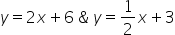Hint:

## The correct answer is: Both are nor parallel neither perpendicular.

### We have been given two equations in the question for which we have to tell are graphs of the equations parallel, perpendicular or neither. Step 1 of 1:We have given two equations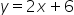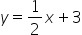Slope of both lines are 2,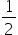respectivelySince slope are not equal then both are not parallel.Product of both slope is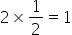So, both are not perpendicular also.So,Both are nor parallel neither perpendicular.#### With Turito Foundation.#### Get an Expert Advice From Turito.